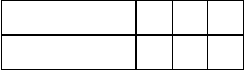Class Notes (1,100,000)
CA (620,000)
UW (20,000)
MATH (2,000)
MATH135 (400)
Lecture 16

# MATH135 Lecture 16: Exercises - Congruence

Department
Mathematics
Course Code
MATH135
Professor
Roxane Itier Itier
Lecture
16

This preview shows pages 1-2. to view the full 8 pages of the document.
MATH 135, Winter 2015
Exercises: Congruence
Note: Unless otherwise speciﬁed, mdenotes a ﬁxed positive integer.
Part 1
1. Verify each of the following statements:
(a) 11 1pmod 2q(e) 4 1004 pmod 10q
(b) 7 22 pmod 5q(f) 4  4pmod 10q
(c) 1241pmod 5q(g) 91 0pmod 13q
(d) 34 1pmod 41q(h) 991234 99 pmod 100q.
2. What time does a clock read:
(a) 29 hours after it reads 11 o’ clock?
(b) 100 hours after it reads 2 o’ clock?
(c) 50 hours before it reads 6 o’ clock?
3. For which mPNare the following statements true?
(a) 27 5pmod mq
(b) 1000 1pmod mq
(c) 1331 0pmod mq
4. Let m, n PNand a, b, c, d PZ. Are the following statements true or false? Explain.
(a) If abpmod mqand cdpmod nq, then acbdpmod mnq.
(b) If abpmod mqand cdpmod nq, then ac bd pmod mnq.
(c) If abpmod mq, then a2b2pmod m2q.
(d) If a, b ¡0 and abpmod mq, then 2a2bpmod mq.
5. (a) What is the last digit of 7535 in its base-10 representation?
(b) What are the last two digits of 7535 in its base-10 representation?
1

Only pages 1-2 are available for preview. Some parts have been intentionally blurred.6. What is the remainder when:
(a) 5099 is divided by 7?
(b) 5099 is divided by 17?
(c) 37100 is divided by 28?
(d) 1060 is divided by 49? (Suggestion: Reduce 210 1024 modulo 49.)
7. (a) Show that 7!  1pmod 71q.
(b) Find the remainder when 70 69 68 67 66 65 64 63 62 is divided by 71.
(Hint: xxmpmod mq.)
8. Show that p6!q2 1pmod 13qand p9!q6 1pmod 13q.
9. Prove that 13 | p270 370 q. (Avoid using Fermat’s Little Theorem.)
10. Show that 7 | p32n12n2qfor all integers n¥0. (Avoid induction.)
11. (a) Given any integer n, evaluate n2pmod 3qaccording to the congruence class (mod 3) of
n. In particular, complete the following table using the integers 0,1,2:
npmod 3q0 1 2
n2pmod 3q
(b) Using (a), prove that for any two integers xand yif 3 -xand 3 -y, then x2y2is not
a perfect square.
12. Using ideas similar to the previous exercise, prove that the sum of two odd perfect squares
can never be a square.
13. Prove that if an integer nis a sum of two squares, then n3pmod 4q. (In other words, any
number of the form 4k3 is not a sum of two squares.)
14. Suppose that a,band k¥1 are integers such that gcdpa, mq  1, akbkpmod mqand
ak1bk1pmod mq. Show that abpmod mq. Is the conclusion still true if the condition
gcdpa, mq  1 is dropped?
15. Using Question 3(a) of Assignment 5, prove that if m, n PNare coprime, then for any x, y PZ,
xypmod mnqif and only if xypmod mqand xypmod nq.
Remark. This result is frequently used in conjunction with the Chinese Remainder theorem.
2
###### You're Reading a Preview

Unlock to view full version

Only pages 1-2 are available for preview. Some parts have been intentionally blurred.

16. Let fpxq  c0c1x. . .cnxnbe a polynomial function with integer coeﬃcients c0, c1, . . . , cn.
Use the basic properties of congruence to prove that for any a, b PZ,
if abpmod mq, then fpaq  fpbq pmod mq.
Remark. To solve the congruence
fpxq  0pmod mq()
means that we seek all integers asuch that fpaq  0pmod mq; any such ais called a solution
to the congruence (). The above result says that if ais a solution to (), then so is every
member bof the congruence class rasm. This justiﬁes calling xapmod mq(or simply
rasm) a single solution. The number of solutions to the congruence () is the number of
congruence classes in Zmthat solve ().
17. Let fpxqbe a polynomial with integer coeﬃcients, and ﬁx nPN. Prove that if ndivides the
values fpkqas kgoes through nconsecutive integers, then n|fpkqfor all integers k.
18. Apply Question 3 of Assignment 6 to solve the congruence 15x30 pmod 105q.
19. Using the fact that 5 22 110, solve the congruence 22x17 pmod 111q.
20. (Some well-known divisibility tests; see Practice 25.4 (p.202) of the course notes.)
Suppose that a natural number nhas decimal representation pakak1. . . a1a0q10, that is,
nak10kak110k1. . . a1101a0, where ajP t0,1,...,9ufor each index 0 ¤j¤k.
Verify the following congruences:
(a) na1101a0pmod 4q;
(b) na2102a1101a0pmod 8q;
(c) nakak1. . . a1a0pmod 3q;
(d) nakak1. . . a1a0pmod 9q;
(e) na0a1a2a3. . .  p1qkakpmod 11q.
These congruences lead to various methods for checking numerical computations, used since
ancient times. For example, from (d) we deduce the rule called ‘casting out nines’, which says
that a number is divisible 9 if and only if the sum of its digits is divisible by 9. Similarly,
(e) implies that a number is divisible by 11 if and only if the alternating sum of its digits is
divisible by 11. Some examples:
(i) Since 3456 34560pmod 9q, we see that 9 |3456.
(ii) Since 120351 1532010pmod 11q, we see that 11 |120351.
3
###### You're Reading a Preview

Unlock to view full version